# Frequency Table Worksheet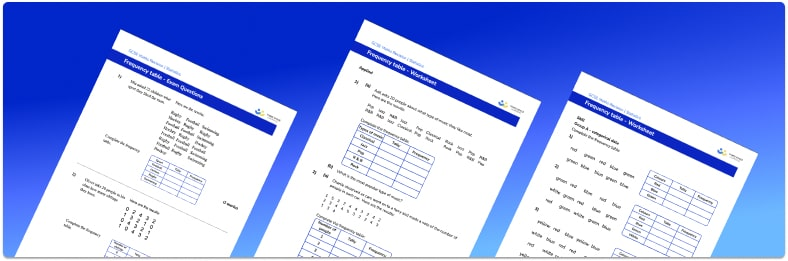Help your students prepare for their Maths GCSE with this free frequency table worksheet of 20 questions and answers

• Section 1 of the frequency table worksheet contains 12 skills-based frequency table questions, in 3 groups to support differentiation
• Section 2 contains 4 applied frequency table questions with a mix of worded problems and deeper problem solving questions
• Section 3 contains 4 foundation and higher level GCSE exam style questions on frequency tables
• Answers and a mark scheme for all frequency table questions are provided
• Questions follow variation theory with plenty of opportunities for students to work independently at their own level
• All questions created by fully qualified expert secondary maths teachers

Suitable for GCSE maths revision for AQA, OCR and Edexcel exam boards

• To receive this resource and regular emails with more free resources, blog posts and other Third Space updates, enter your email address and click below.

• This field is for validation purposes and should be left unchanged.

You can unsubscribe at any time (each email we send will contain an easy way to unsubscribe). To find out more about how we use your data, see our privacy policy.

### Frequency table at a glance

A frequency table is used to display a data set; it is often the starting point for graphing frequency diagrams such as bar charts, pictograms and histograms. The first column in a frequency table shows the data values – categorical or quantitative. The second column is the frequency of each data value. For example, a frequency table might display the number of goals scored by a football team over a given number of matches – in this case, the first column would have the number of goals (0, 1, 2, 3,…).

When constructing a frequency table, it is often useful to begin with a tally chart to organise and count the number of data items in each category or group.

We can easily find the mode from a frequency table by identifying the category or group with the highest frequency

To find the mean for ungrouped data, we multiply the data value by its frequency for each category, then sum and divide by the number of pieces of data. To estimate the mean for grouped data, we use the same process as above, using the midpoint of each group to estimate the data value.

Looking forward, students can then progress to additional Statistics worksheets, for example a mean, median, mode and range worksheet or pie chart worksheet.For more teaching and learning support on Statistics our GCSE maths lessons provide step by step support for all GCSE maths concepts.

## Related worksheets

Types Of Data Worksheet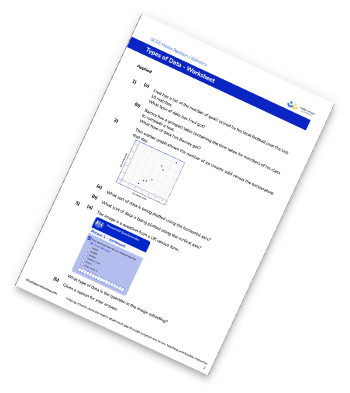X And Y Axis Worksheet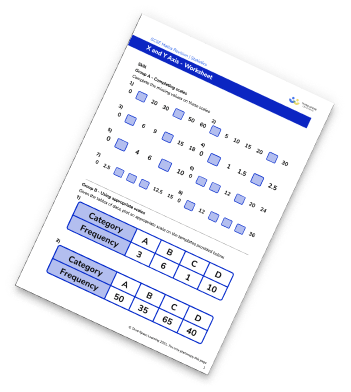Tally Charts Worksheet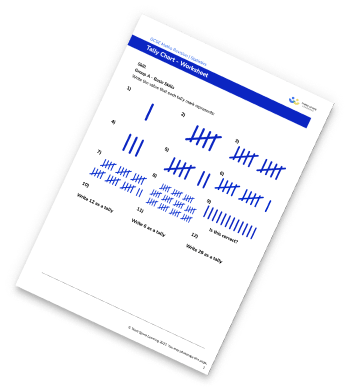Quartile And Interquartile Range Worksheet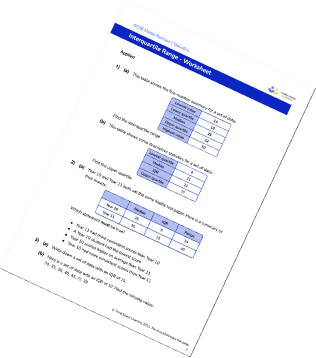## Do you have KS4 students who need more focused attention to succeed at GCSE?There will be students in your class who require individual attention to help them succeed in their maths GCSEs. In a class of 30, it’s not always easy to provide.

Help your students feel confident with exam-style questions and the strategies they’ll need to answer them correctly with our dedicated GCSE maths revision programme.

Lessons are selected to provide support where each student needs it most, and specially-trained GCSE maths tutors adapt the pitch and pace of each lesson. This ensures a personalised revision programme that raises grades and boosts confidence.

Find out more